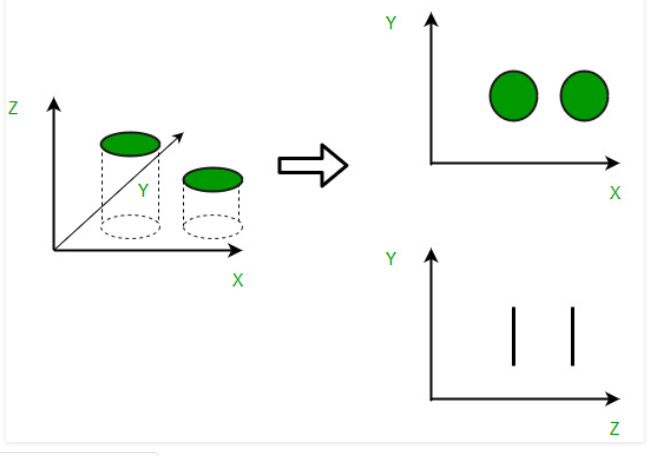2 views

What does dimensionality reduction mean exactly?

I searched for its meaning, I just found that it means the transformation of raw data into a more useful form. So what is the benefit of having data in a useful form, I mean how can I use it in practical life (application)?

by (108k points)

Dimensionality reduction is the process of reducing the number of random variables of the program under consideration, by obtaining a set of principal variables. For example,  a simple email classification problem, where we need to classify whether the email is spam or not. This can involve a large number of features, such as whether or not the e-mail has a generic title, the content of the e-mail, whether the email uses a template, etc. Here is the diagrammatic representation of dimensionality reduction:It can be divided into:

• Feature selection:  We find a subset of the original set of variables, or features, to get a smaller subset that can be used to model the problem. It usually involves three ways:

1. Filter

2. Wrapper

3. Embedded

• Feature extraction: This reduces the data in a high dimensional space to a lower dimension space, i.e. a space with lesser no. of dimensions.

Dimensionality reduction is a Machine Learning Technique of diminishing the measure of Random Variables in a Problem by acquiring a lot of head Variables. So for more insights about Dimensionality reduction visit this Machine Learning Course.